# Chapter 10 Review of R and New tricks

By now, we have hopefully gotten the hang of getting things done in R. But we’re all in a different place. Some of us still have some fundamental things we don’t understand. Others feel ok on the whole, but there might be something that you don’t quite get, or a bunch of confusing errors that you haven’t understood.

Maybe you have R questions related to work you have done outside of his class, or you are curious about base R.

Or you want to make nice reproducible reports in RMarkdown and/or learn how to organize an RProject, or organize make a collaborative open project on github.

This lesson is designed to give you a chance to catch up on R, connect the dots between what you have learned, review, and/or learn new things. This should help you prepare for your first major assignment of an exploratory data analysis, due on Monday.

## 10.1 When R goes wrong

R can be fun, useful and empowering, but it can also be a bummer. Sometimes it seems like you’ve done everything right, but things still aren’t working. Other times, you just can’t figure out how to do what you want. When this happens it is natural to tense up, curse, throw $h!t etc… While some frustration is OK, you should try to avoid sulking. Here’s what I do. 1. Take a deep breath. 2. Copy and paste and try again. If that doesn’t work, go to 3. 3. Look at the code for any common issues (like those in Section 10.1.2, below). If I see one of these issues, I fix it and feel like a champ. If not, I go to step 4. 4. Remember that google is my friend, use it. I usually copy the error message R gives and paste it into google. Does this give me a path towards solving this? If so give it a shot, if not, go to 5. 5. Take a walk around the house, grab a drink of water, get away from the computer for a minute. Then think about what I was trying to do and return to the computer to see if I’ve done it right and if that time away allowed me to see mistakes I missed before. If I see the issue, I fix it and feel like a champ. If not, I go to step 6. 6. Move on, do something else and come back to it later (7). If you have more R to work on, try that (unless you need a break). If you’re not going to do more R, you should probably close your RStudio session. 7. OK back to it. Reopen RStudio and work through your code. Do you see the issue now? If so fix it, if not move onto 8. 8. Explain the issue to a friend / peer. I often figure out what I did wrong, when I explain it. Like “I said add 5+5 and it kept giving me 10, when the answer should have been 25.” And then I realize I added when I wanted to multiply. Or maybe your friend figures it out. 9. How important is this thing? Can I do something slightly different that is good enough? If so I try that. If it’s essential, 10. Find an expert or stackoverflow or something. #### 10.1.0.1 Run only a few lines When you type more complex stuff errors are bound to show up somewhere. I suggest running each line one at a time (or at least running a few lines at a time to unstick yourself if you found an error 10.1). include_graphics("images/run a few lines.jpeg")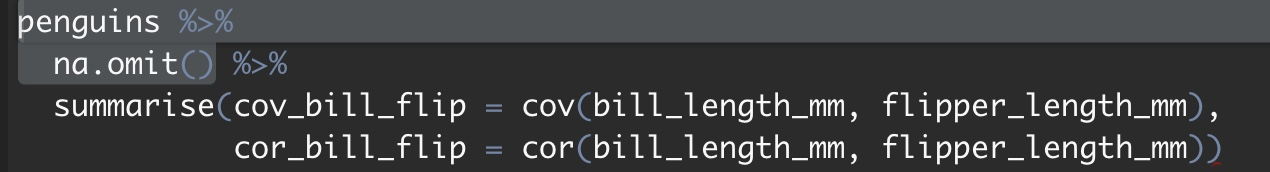Figure 10.1: You can run a few lines by highlighting what you want to turn (make sure not to end on a pipe %>%). ### 10.1.1 Warnings and Errors, Mistakes and Impasses Before digging into the common R errors, lets go over the four ways R can go wrong. 1. A warning. We did something that got R nervous, and it gives us a brief message. It is possible everything is ok, but just have a look. I think of this as a yellow light. The most common warning I get is the harmless summarise() ungrouping output (override with .groups argument). 2. An error. We did something that R does not understand or cannot do. R will stop working and make us fix this. I think of this as a red light. 3. A mistake. Our communication with R broke down – it thought we were doing one thing but we wanted it to do another. Mistakes are the most likely to cause a big problem. So, remember that just because R works without an error or warning, does not mean it did what we hoped. 4. An impasse. There’s something we want to do, but can’t figure it out. Be mindful of these types of issues with R as you code and as you read the common errors below. ### 10.1.2 Common gotcha’s I share my most common mistakes, below. I note that these are my common mistakes. If you find that you often make different sorts of mistakes, email me with them, and I’ll add them. #### 10.1.2.1 Spelling / capitilization etc R cant read your mind (although tab-completion in RStudio is awesome), and pays attention to capital letters. Spellng errors are my most common mistake. For example, the column, Sepal.Length, in the iris dataset has sepal lengths for a bunch of individuals. Trying to select the column by typing any one of the options below will yield a similar error: dplyr::select(iris, sepal.length) dplyr::select(iris, Sepal_Length) dplyr::select(iris, Sepal.Lngth) ## Error: Can't subset columns that don't exist. ## x Column Sepal.Lngth doesn't exist. Similarly, you might misspell the function: dplyr::selct(iris, Sepal.Length) ## Error: 'selct' is not an exported object from 'namespace:dplyr' So, check for these mistakes and fix the code above to look like this: dplyr::select(iris, Sepal.Length) ## [38;5;246m# A tibble: 6 x 1[39m ## Sepal.Length ## [3m[38;5;246m<dbl>[39m[23m ## [38;5;250m1[39m 5.1 ## [38;5;250m2[39m 4.9 ## [38;5;250m3[39m 4.7 ## [38;5;250m4[39m 4.6 ## [38;5;250m5[39m 5 ## [38;5;250m6[39m 5.4 When it comes to spelling errors, a helpful hint might be to have a consistent (As possible) way of naming vectors, eg. always_separate_with_underscores, or AlwaysCapitalizeTheFirstLetter, or always.separate.with.periods #### 10.1.2.2 Confusing == and = Consecutive equals signs == ask if the thing on the right equals the thing on the left. For example, if I want to know if two equals six divided by two, I type 2 == (6/2) and R says FALSE. But what if I accidentally type (6/2) = 3. In this case R gets very confused.  2 = (6/2) ## Error in 2 = (6/2): invalid (do_set) left-hand side to assignment This confusion arises because we told R to make two equal six divided by two, which is nonsense. two <- 2 But it could be worse than nonsense. Say the value two is assigned to two, and now we ask if two equals (6/2). Asking with a ==, as in two == (6/2), gives us our expected answer: FALSE. But typing two = 6/2 does not ask if two equals 6/2, rather it tells R that two equals 6/2 for now, returning unexpected results like that below. two^2 ##  9 This is one of many cases in which R does what we say and does not warn of an error, but does not do what we hoped it would. One more note while we’re here: The clarity and utility of R’s error messages vary tremendously. For example, if we confuse = and == inside the filter() function in the dplyr package, R gives a very useful error message. For example, say we mess up in asking R to only return data for Iris setosa from the iris data set. filter(.data = iris, Species = "setosa") ## Error: Problem with filter() input ..1. ## x Input ..1 is named. ## ℹ This usually means that you've used = instead of ==. ## ℹ Did you mean Species == "setosa"? By contrast doing a similar operation in base R (which you may have seen previously, but we don’t cover in this course) , yields a less clear error message: iris[ iris$Species = "setosa", ]
## Error: <text>:1:20: unexpected '='
## 1: iris[ iris$Species = ## ^ #### 10.1.2.3 Confusing = and <- As we’ve seen throughout the course, we use the global operator, to assign values to variables. But inside a function we use the = sign to assign values to argument in a function. Using the global operator in a function does a bunch of bad things. Say we wanted to sample a letter from the alphabet at random. Typing sample(x = letters, size =1), will do the trick and will not assign any value to x outside of that function. #### DO THIS sample(x = letters[1:10], size =1) ##  "d" x ## Error in eval(expr, envir, enclos): object 'x' not found The error above is a good thing – we wanted to sample letters, not have x equal the letters. By contrast, using <- to assign values to arguments has bad consequences. #### DONT DO THIS sample(x <- letters, size =1) ##  "i" x ##  "a" "b" "c" "d" "e" "f" "g" "h" "i" "j" "k" "l" "m" "n" "o" "p" "q" "r" "s" "t" "u" "v" "w" ##  "x" "y" "z" This is BAD. We did not want to assign the alphabet to x, we just wanted the sample() function to sample from the alphabet. Bottom line: • Use the = sign to assign values to arguments in a function. • Use <- to assign values to variables outside of a function. • Use == to ask if one thing equals another. #### 10.1.2.4 Dealing with missing data Often our data includes missing values. When we do math on a vector including missing values, R will return NA unless we tell it to do something else. See below for an example: my_vector <- c(3,1,NA) mean(my_vector) ##  NA Depending on the function, we have different ways of telling R what to do with missing data. In the mean() function, we type. mean(my_vector, na.rm = TRUE) ##  2 We have to be extra careful of missing values when doing math ourselves. If we found the mean of my_vector by dividing its sum by its length, like this: sum(my_vector) / length(my_vector) = NA, we would have the wrong answer. So be careful and avoid this mistake. #### 10.1.2.5 Conflicts in function names Each function in a package must be unique. But functions in different packages can have the same name and do different things. This means we might be using a totally different function than we think. If we’re lucky this results in an error, and we can fix it. If we’re unlucky, this results in a bad mistake. We can avoid this mistake by typing the package name and two colons and then the function name (e.g. dplyr::filter) before using any function. But this is quite tedious. Installing and loading the conflicted package, which tells us when we use a function that is used by more than one package loaded, resulting in a warning that we can fix! #### 10.1.2.6 Mistakes in assignment I often mess up in assigning values to variables. I do so in a few different ways, I: • Forget to assign a variable to memory, • I use the variable before it’s assigned, • I don’t update my assignment after doing something, or • I overwrite my old assignment. I’ll show you what I mean and how to spot and fix these common issues… ##### 10.1.2.6.1Mistakes in assignment: Not in memory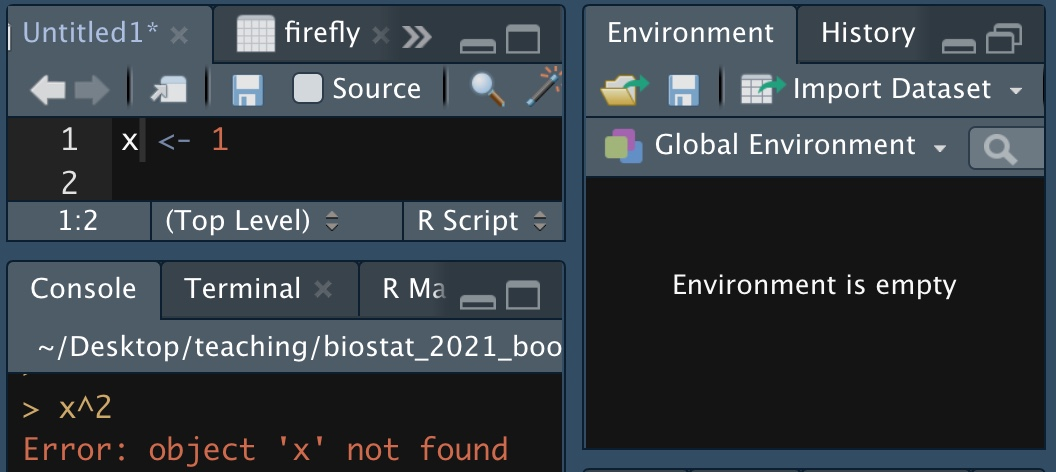Figure 10.2: I did not assign the value one to x. As the example in Figure 10.2 shows, typing a value into an R script is not enough. We need to enter it into memory. You can do this by either, hitting ctrl + shift, or /command + shift, or hitting the run button in the RStudio IDE, or copying and pasting into the terminal widow. To see all the variables in Rs memory, check the environment tab in the RStudio IDE, or use the ls() function with no arguments. ##### 10.1.2.6.2Mistakes in assignment: Wrong order If you want to square x, (as in Figure 10.2), you must assign it a value first. This mistake is quite similar to the one above. x^2 x <- 1 ## Error in x^2: non-numeric argument to binary operator ##### 10.1.2.6.3Mistakes in assignment: Not updating assignment Another common mistake is to run some code but not assign the output to anything. So, for example we wanted to make a density plot of the ratio of petal width to sepal width. Can you spot the error in the code below? iris %>% mutate(petal_to_sepal_width = Petal.Width / Sepal.Width)  ## [38;5;246m# A tibble: 150 x 6[39m ## Sepal.Length Sepal.Width Petal.Length Petal.Width Species petal_to_sepal_width ## [3m[38;5;246m<dbl>[39m[23m [3m[38;5;246m<dbl>[39m[23m [3m[38;5;246m<dbl>[39m[23m [3m[38;5;246m<dbl>[39m[23m [3m[38;5;246m<fct>[39m[23m [3m[38;5;246m<dbl>[39m[23m ## [38;5;250m 1[39m 5.1 3.5 1.4 0.2 setosa 0.057[4m1[24m ## [38;5;250m 2[39m 4.9 3 1.4 0.2 setosa 0.066[4m7[24m ## [38;5;250m 3[39m 4.7 3.2 1.3 0.2 setosa 0.062[4m5[24m ## [38;5;250m 4[39m 4.6 3.1 1.5 0.2 setosa 0.064[4m5[24m ## [38;5;250m 5[39m 5 3.6 1.4 0.2 setosa 0.055[4m6[24m ## [38;5;250m 6[39m 5.4 3.9 1.7 0.4 setosa 0.103 ## [38;5;250m 7[39m 4.6 3.4 1.4 0.3 setosa 0.088[4m2[24m ## [38;5;250m 8[39m 5 3.4 1.5 0.2 setosa 0.058[4m8[24m ## [38;5;250m 9[39m 4.4 2.9 1.4 0.2 setosa 0.069[4m0[24m ## [38;5;250m10[39m 4.9 3.1 1.5 0.1 setosa 0.032[4m3[24m ## [38;5;246m# … with 140 more rows[39m ggplot(iris, aes(x = petal_to_sepal_width, fill = Species )) + geom_density(alpha = .5) ## Error in FUN(X[[i]], ...): object 'petal_to_sepal_width' not foundWe clearly calculated petal_to_sepal_width, above, so why can’t R find it? The answer is that we did not save the results. Let’s fix this by assigning our new modifications to iris. iris <- iris %>% mutate(petal_to_sepal_width = Petal.Width / Sepal.Width) ggplot(iris, aes(x = petal_to_sepal_width, fill = Species )) + geom_density(alpha = .5)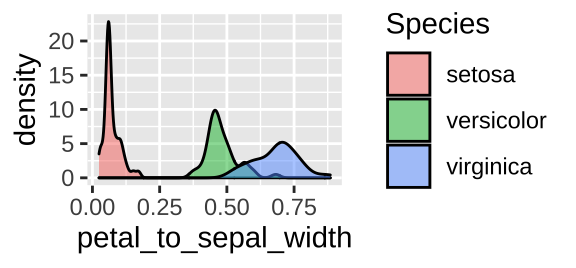##### 10.1.2.6.4Mistakes in assignment: Overwriting assignments Above, I showed how failing to reassign after doing some calculation can get us in trouble. But other times, reassigning can cause it own problems. For example, let’s say I want to calculate mean() petal to sepal widths for each species and then make the same plot as above. iris <- iris %>% group_by(Species) %>% dplyr::summarize(mean_petal_to_sepal_width = mean(petal_to_sepal_width))  ## summarise() ungrouping output (override with .groups argument) ggplot(iris, aes(x = petal_to_sepal_width, fill = Species )) + geom_density(alpha = .5) ## Error in FUN(X[[i]], ...): object 'petal_to_sepal_width' not found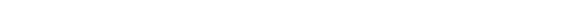So, what went wrong here? Let’s take a look at what I did to iris: iris ## [38;5;246m# A tibble: 3 x 2[39m ## Species mean_petal_to_sepal_width ## [3m[38;5;246m<fct>[39m[23m [3m[38;5;246m<dbl>[39m[23m ## [38;5;250m1[39m setosa 0.071[4m9[24m ## [38;5;250m2[39m versicolor 0.480 ## [38;5;250m3[39m virginica 0.684 Ooops, we just have species means. By combining summarise with a reassignment, we replaced the whole iris dataset with a summary of means. In this case, it’s better to assign your output to a new variable… iris_petal2sepalw_bysp <- iris %>% group_by(Species) %>% dplyr::summarize(mean_petal_to_sepal_width = mean(petal_to_sepal_width), .groups= "drop_last") ggplot(iris, aes(x = petal_to_sepal_width, fill = Species )) + geom_density(alpha = .5)No worries though if you have your code well laid out, you can just rerun everything until the pint before you made this mistake and you’re back in business. So when should we reassign to the old variable name, and when should we assign to a new name? My rule of thumb is to reassign to the same variable when I add things to a tibble, but do not change existing data, while I assign to a new variable when values change or are removed (with some exceptions). But what if you wanted the mean in the same tibble as the initial data so you could add lines for species means? You can so this with mutate() instead of summarise(). iris <- iris %>% group_by(Species) %>% dplyr::mutate(mean_petal_to_sepal_width = mean(petal_to_sepal_width), .groups= "drop_last") ggplot(iris, aes(x = petal_to_sepal_width, fill = Species)) + geom_density(alpha = .5)+ geom_vline(aes(xintercept = mean_petal_to_sepal_width, color = Species), lty = 2)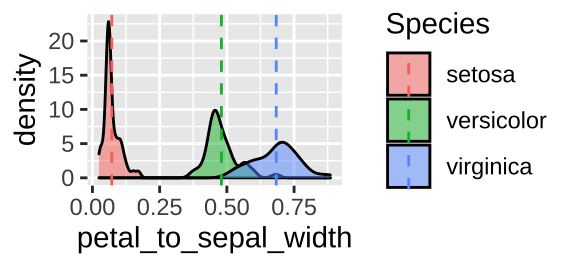#### 10.1.2.7 Just because you didn’t get an error doesn’t mean R is doing what you want (or think): Say you want to reassign x to the value 10, and you want y to equal x$^2\$ (so y should be 100). The code below messes this up by assigning the value x^2 to y before it setting x to 10. This means that y is using the older value x, which equals 1, set above.

y  <-   x^2
x  <-   10
print(y)
##  1

#### 10.1.2.8 Unbalanced parentheses, quotes, etc…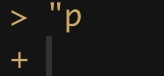Some things, like parentheses and quotes come in pairs. Too many or too few will cause trouble.• If you have one quote or parenthesis open, without its partner (e.g. "p), R will wait for you to close it. With a short piece of code you can usually figure out where to place the partner. If the code is long and it’s not easy to spot, hit escape to start that line of code over.

• Also, in a script, clicking the space after a parenthesis should highlight its partner and bring up a little X in the sidebar to flag missing parentheses.

• Unbalanced parentheses cause R to report an error (below).

c((1)
## Error: <text>:2:0: unexpected end of input
## 1: c((1)
##    ^

#### 10.1.2.9 Common issues in ggplot

##### 10.1.2.9.1 Using %>% instead of +

We see that tidyverse has two ways to take what it has and keep moving.

• When dealing with data, we pipe opperations forward with the %>% operator. For example, we told R to tak our tibble and then pull out our desired coumn, above name_of_tibble %>% pull(var = name_of_column).

• When building a plot, we add elements to it with the + sign. For example, in a scatterplot, we type: ggplot(data = <name_of_tibble>, aes(x=x_var, y = y_var)) + geom_point().

I occasionally confuse these and get errors like this:

ggplot(iris, aes(x = Petal.Length, y = Petal.Width)) %>%
geom_point()
## Error: mapping must be created by aes()
## Did you use %>% instead of +?

Or

iris +
summarise(mean_petal_length = mean(Petal.Length))
## Error in h(simpleError(msg, call)): error in evaluating the argument 'x' in selecting a method for function 'mean': object 'Petal.Length' not found
##### 10.1.2.9.2 Specifying a color in the aes argument

Say we want to plot the relationship between petal and sepal length in Iris setosa with points in blue.

The code below is a common way to do this wrong.

filter(iris, Species == "setosa") %>%
ggplot(aes(x=Petal.Length, y = Sepal.Length, color = "blue")) +
geom_point()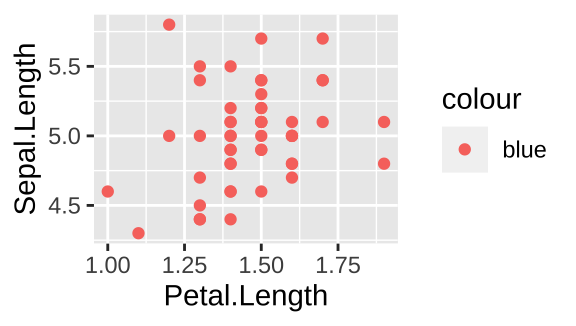The right way to do this is

filter(iris, Species == "setosa") %>%
ggplot(aes(x=Petal.Length, y = Sepal.Length)) +
geom_point(color = "blue")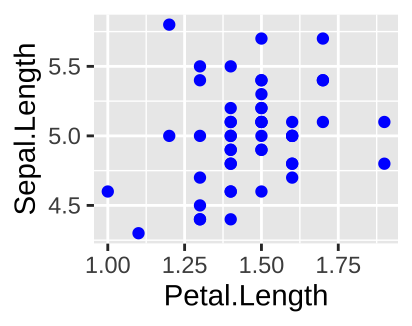##### 10.1.2.9.3 Fill vs color

There are two types of things to color in R – the fill argument fill space with a color and the color argument colors lines and points. To demonstrate let’s first make two histograms of Iris setosa sepal length with decorative color: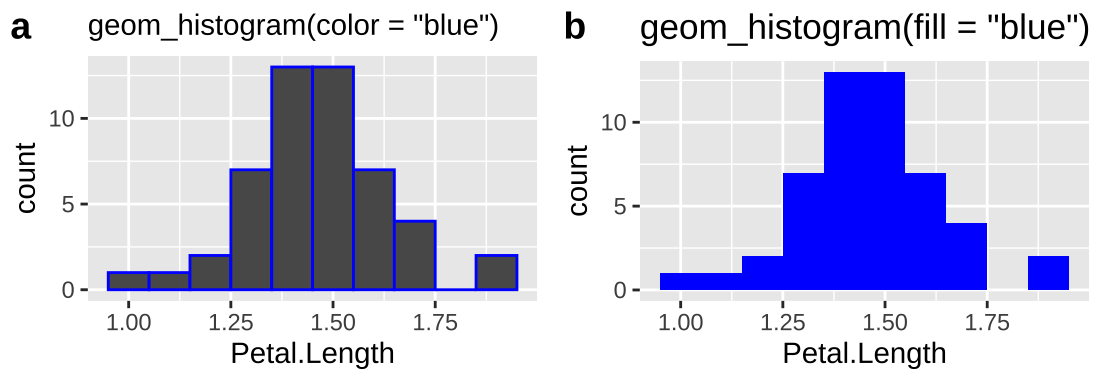So we usually want to make histograms and density plots by specifying our desired color with fill. I usually add color = "white" to separate the bars in the histogram.*

Now let’s try the same for color, now plotting petal length against sepal length, mapping species onto color or fill.

plotA <- ggplot(iris, aes(x=Petal.Length,  y = Sepal.Length, color = Species)) +
geom_point()+   labs(title = 'aes(color = Species)')

plotB <- ggplot(iris, aes(x=Petal.Length, y = Sepal.Length, fill = Species)) +
geom_point()+  labs(title = 'aes(fill = Species)')

# using plot_grid in the cowplot package to combine plots
plot_grid(plotA, plotB, labels = c("a","b"))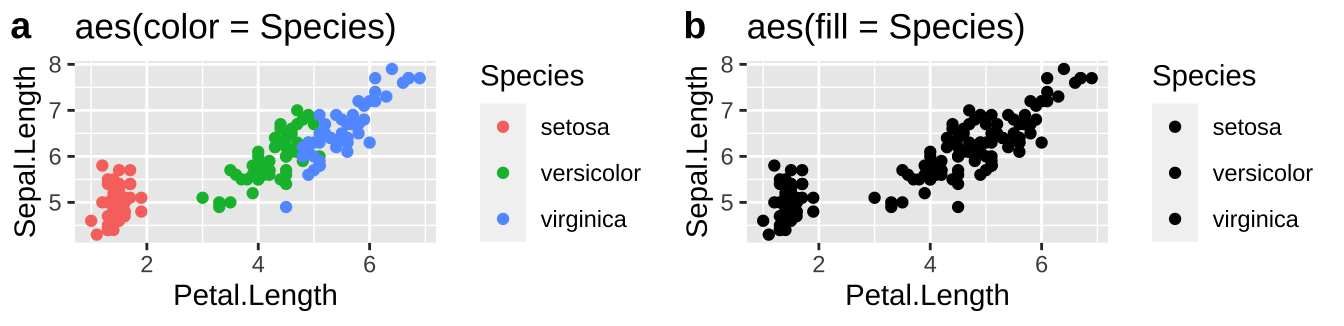So we usually want to make scatterplots and lineplots by specifying our desired color with color.

##### 10.1.2.9.4 Bar plots with count data

Imagine we wanted to plot hair color and eye color for men and women. Because this is count data, some form of bar chart would be good. If our data looks like this

We can make a bar plot with geom_bar

ggplot(hair_eye, aes(x = Sex, fill = Eye))+
geom_bar(position = "fill", color = "white")+
facet_wrap(~Hair, nrow = 1, labeller = "label_both")+
scale_fill_manual(values = c("blue","brown","green","gold"))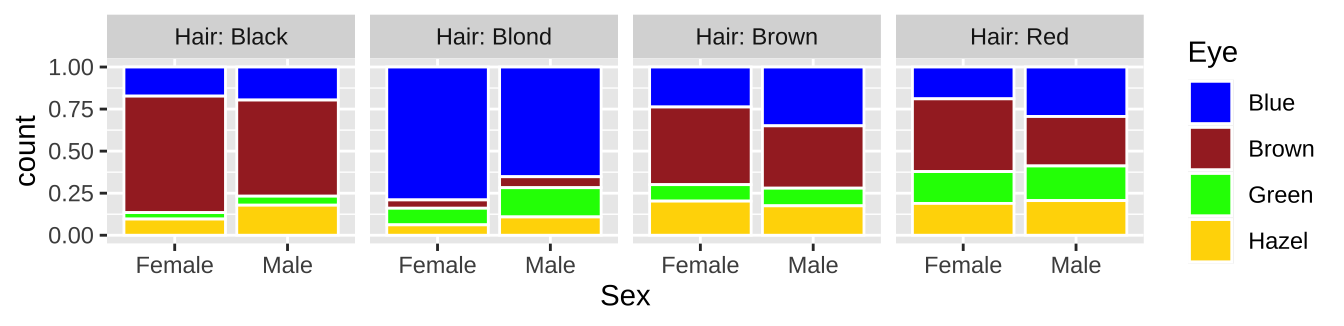But if our data looked like this:

geom_bar would result in an error.

ggplot(HairEyeColor, aes(x = Sex, y = n, fill = Eye))+
geom_bar(position = "fill", color = "white")+
facet_wrap(~Hair, nrow = 1, labeller = "label_both")+
scale_fill_manual(values = c("blue","brown","green","gold"))
## Error: stat_count() can only have an x or y aesthetic.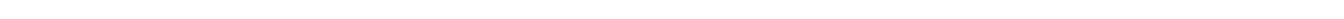We could overcome this error by using geom_col() instead of geom_bar(), or by typing geom_bar(position = "fill", color = "white", stat = 'identity').

#### 10.1.2.10 When you give R a tibble

Throughout this course, we deal mostly with data in tibbles, a really nice way to store a bunch of variables of different classes – each as its own vector in a column. However occasionally we need to pull a vector from its tibble, to do so, type:

pull(.data = <name_of_tibble>, var = <name_of_column>)
## or
name_of_tibble %>%
pull(var = name_of_column)

## 10.2 Enhancing your R experience

### 10.2.1 RMarkdownRMarkdown is a file format that allows us to seamlessly combine text, R Code, results and plots. You use RMarkdown by writing in plain text and then interspersed with code chunks. See the video below (10.3) for a brief overview.

Figure 10.3: A brief (4 min and 37 sec) overview of RMarkdown from Stat 545.

You can use RMarkdown to make pdfs, html, or word documents that you can share with peers, employers etc… RMardown is especially useful for communicating results of complex data analysis, as your numbers, figures, and code will all match. This also means that anyone (especially future you, See Fig. 10.4) can recreate, learn from, and build off of your work.

Figure 10.4: Why make a reproducible workflow (A dramatic 1 min and 44 sec video).

Many students in this course like to turn in their homeworks as html documents generated by RMarkdown, because they can share their code, figures and ideas all in one place. Outside of class, the benefits are similar – people can see your code and results as they read your explanation. RMarkdown is pretty flexible – you can write lab reports, scientific papers, or even this book in RMarkdown.

To get started with RMarkdown, I suggest click File > New File > RMarkdown and start exploring. For a longer introduction, check out Chapter 27 of R for Data Science . Push onto Chapter 2 of RMarkdown: The definitive guide to dig even deeper.

A few RMarkdown tips:

• You can control figure size by specifying fig.height and fig.width and you can show the code or not with the echo = TRUE or echo = FALSE options in the beginning of your codechunk {r, fig.height = ..., fig.width = ..., echo = ...}).

• The DT and kableExtra packages can help make prettier tables.

If you have the time and energy, I strongly recommend that you turn in your first homework as an html generated by RMarkdown.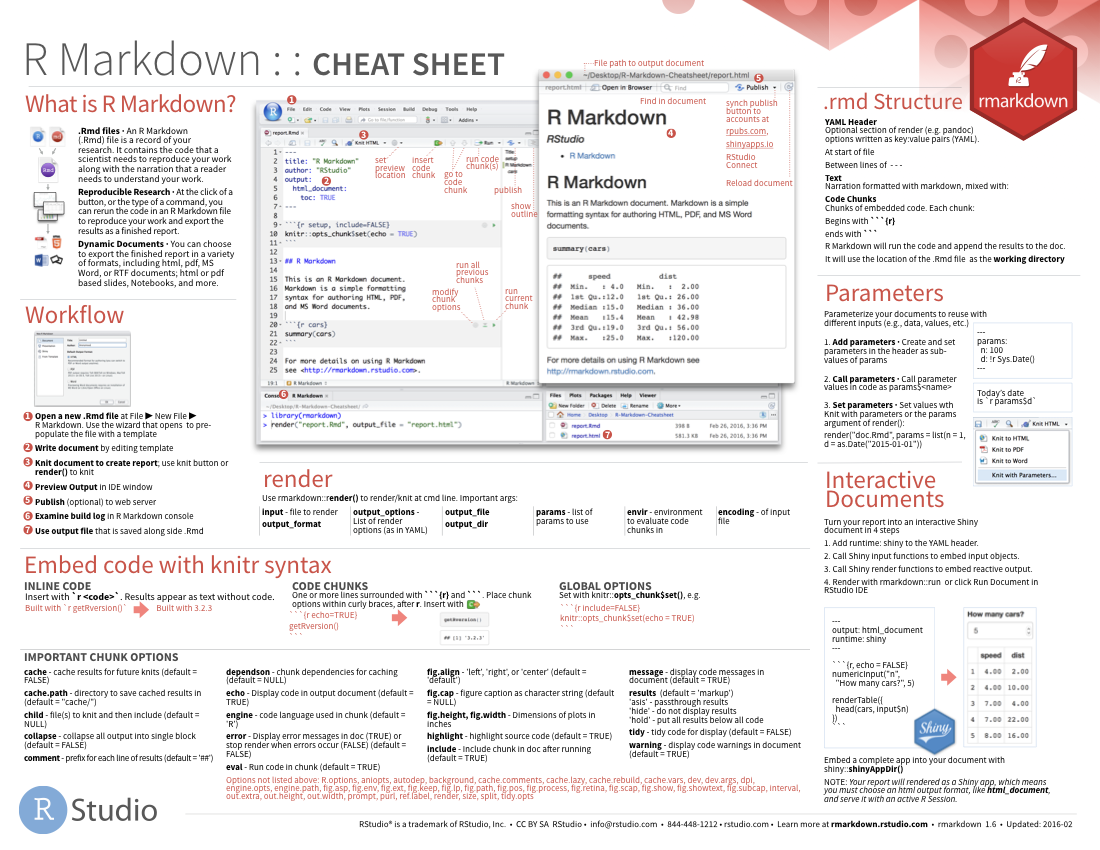Figure 10.5: download the RMarkdown cheat sheet

## 10.3 Your questions, answered

The most common questions where about plotting and summarizing data. I’ll review quickly.

### 10.3.1 Using ggplot: Which type of plot? + summarizing data

Let’s revisit our Palmer penguins data

library(palmerpenguins)

#### 10.3.1.1 One categorical variable

Say we wanted to know how many penguins came from each island. We could visualize this with a barplot:

ggplot(penguins, aes(x = island)) +
geom_bar( fill = "black")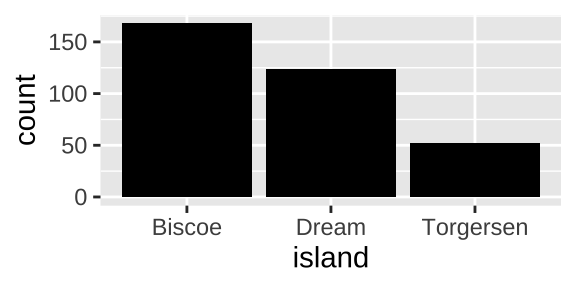and quantify our answers by summarizing, grouping by island and finding n(),

penguin_islands <- penguins %>%
group_by(island) %>%
summarise(count= n())

penguin_islands
## [38;5;246m# A tibble: 3 x 2[39m
##   island    count
##   [3m[38;5;246m<fct>[39m[23m     [3m[38;5;246m<int>[39m[23m
## [38;5;250m1[39m Biscoe      168
## [38;5;250m2[39m Dream       124
## [38;5;250m3[39m Torgersen    52

If our data started out summarized like this we would make a barplot with geom_col()

ggplot(penguin_islands, aes(x = island, y = count)) +
geom_col(fill = "black")#### 10.3.1.2 Two categorical variables

Say we wanted to know how many penguins came from each island for each speies. We could visualize this with a barplot:

ggplot(penguins, aes(x = island, fill = species)) +
geom_bar(position = position_dodge(preserve = "single"))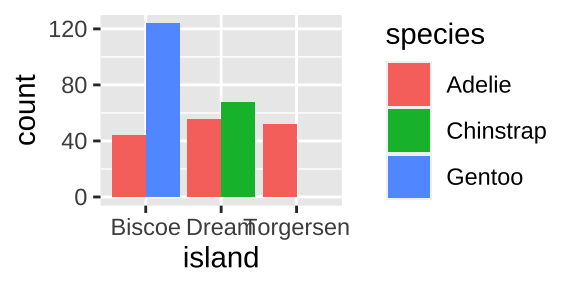again we quantify our answers by summarizing, grouping by island and species and finding n(),

penguins %>%
group_by(island, species, .drop = FALSE) %>%
summarise(count= n())
## [38;5;246m# A tibble: 9 x 3[39m
## [38;5;246m# Groups:   island [39m
##   island    species   count
##   [3m[38;5;246m<fct>[39m[23m     [3m[38;5;246m<fct>[39m[23m     [3m[38;5;246m<int>[39m[23m
## [38;5;250m1[39m Biscoe    Adelie       44
## [38;5;250m2[39m Biscoe    Chinstrap     0
## [38;5;250m3[39m Biscoe    Gentoo      124
## [38;5;250m4[39m Dream     Adelie       56
## [38;5;250m5[39m Dream     Chinstrap    68
## [38;5;250m6[39m Dream     Gentoo        0
## [38;5;250m7[39m Torgersen Adelie       52
## [38;5;250m8[39m Torgersen Chinstrap     0
## [38;5;250m9[39m Torgersen Gentoo        0

#### 10.3.1.3 One continuous variable

Say we want to look at the distribution of one variable like, bill length. A histogram or density plot would be best. For both visualizations, x refers to a quantitative value and y shows how frequent his value is. The histogram counts all values in a given bin on the x, while the density plot fits a curve t interpolate.

ggplot(penguins, aes(x = bill_length_mm)) +
geom_histogram(bins = 30, fill = "black", color  = "white")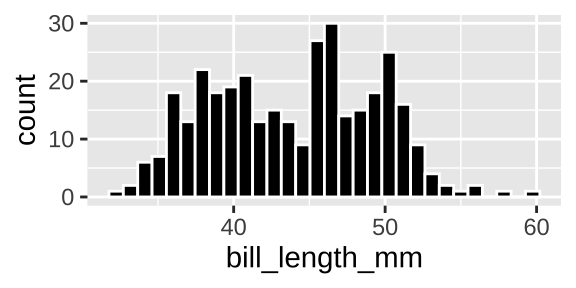ggplot(penguins, aes(x = bill_length_mm)) +
geom_density(adjust = .5, fill = "black")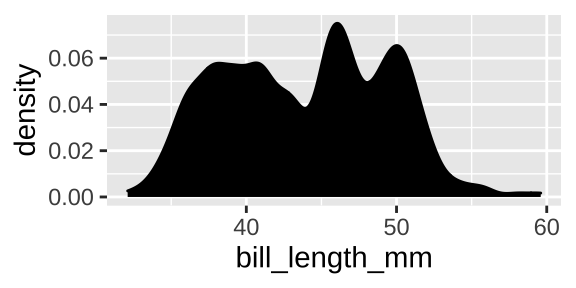We could summarise the sample size, sample mean, and sample standard deviation filtering for only cases with non-missing bill length data.

penguins  %>%
filter(!is.na(bill_length_mm)) %>%
summarise(n = n(),
mean_bill_length = mean(bill_length_mm),
sd_bill_length = sd(bill_length_mm))
## [38;5;246m# A tibble: 1 x 3[39m
##       n mean_bill_length sd_bill_length
##   [3m[38;5;246m<int>[39m[23m            [3m[38;5;246m<dbl>[39m[23m          [3m[38;5;246m<dbl>[39m[23m
## [38;5;250m1[39m   342             43.9           5.46

#### 10.3.1.4 One categorial and one continuous variable

Say we wanted to show the bill length distribution for each species. A jitter plot is good at highlighting differences in mean and distribution.

ggplot(penguins, aes(x = species, y = bill_length_mm, color = species)) +
geom_jitter(height = 0, width = .2, alpha = .4, show.legend = FALSE)+
stat_summary(fun.data = "mean_cl_boot", color = "black")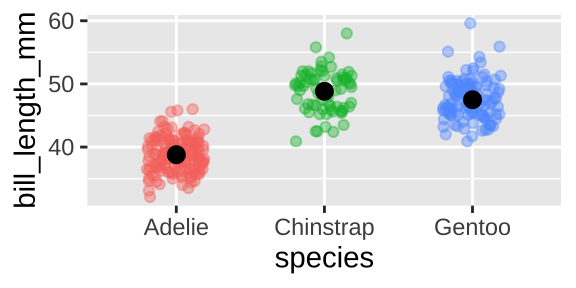Some people might like a boxplot which highlights the quartiles of our data, but I’m less into them, and when I use them I combine with a jitterplot

ggplot(penguins, aes(x = species, y = bill_length_mm, color = species)) +
geom_boxplot(color = "black", outlier.colour = NA)+
geom_jitter(height = 0, width = .2, alpha = .4, show.legend = FALSE)+
stat_summary(fun.data = "mean_cl_boot", color = "black")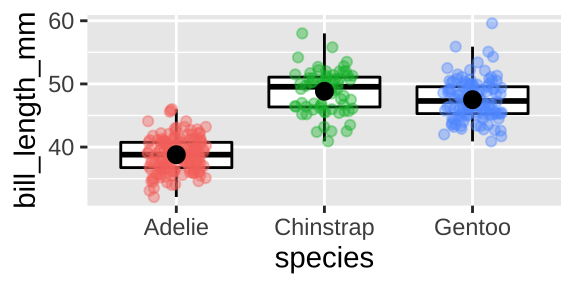If you were more focused on the distribution than the mean, a density plot would make the distribution easier to visualize

ggplot(penguins, aes(x = bill_length_mm, fill = species)) +
geom_density(alpha = .4)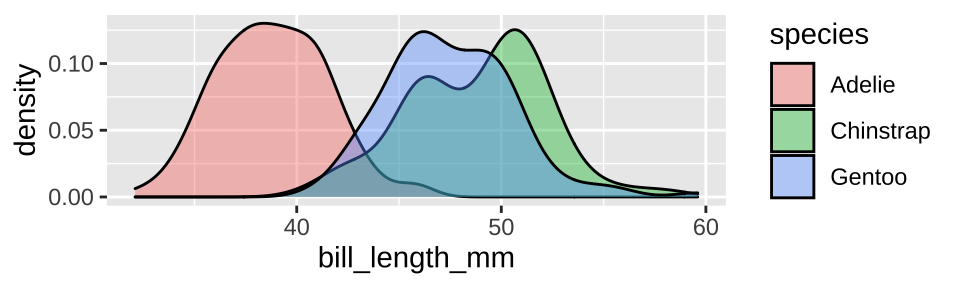Alternatively a histogram cold function similarly – but we should facet by species and make sure there’s only one column so it’s easy to compare

ggplot(penguins, aes(x = bill_length_mm, fill = species)) +
geom_histogram(color = "white", show.legend = FALSE)+
facet_wrap(~species, ncol =1)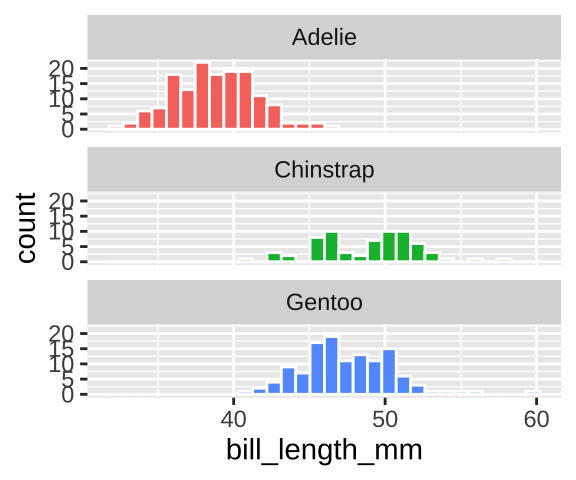We could summarize within species by using a group_by()

penguins  %>%
filter(!is.na(bill_length_mm))%>%
group_by(species) %>%
summarise(n = n(),
mean_bill_length = mean(bill_length_mm),
sd_bill_length = sd(bill_length_mm))
## [38;5;246m# A tibble: 3 x 4[39m
##   species       n mean_bill_length sd_bill_length
##   [3m[38;5;246m<fct>[39m[23m     [3m[38;5;246m<int>[39m[23m            [3m[38;5;246m<dbl>[39m[23m          [3m[38;5;246m<dbl>[39m[23m
## [38;5;250m1[39m Adelie      151             38.8           2.66
## [38;5;250m2[39m Chinstrap    68             48.8           3.34
## [38;5;250m3[39m Gentoo      123             47.5           3.08

#### 10.3.1.5 Two continuous variables

Say we wanted to show the association between bill length and flipper length. A scatterplot is appropriate.

ggplot(penguins, aes(x = bill_length_mm, y = flipper_length_mm)) +
geom_point()+
geom_smooth(method = "lm", se = FALSE)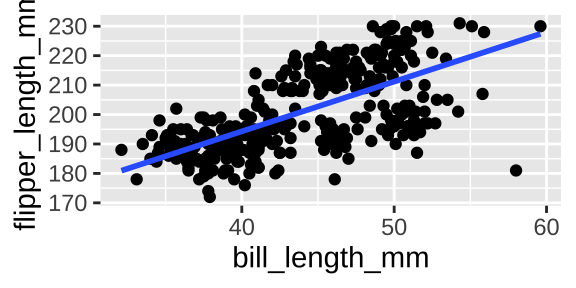And we could summarize this association with the covariance or correlation

penguins %>%
na.omit() %>%
summarise(cov_bill_flip = cov(bill_length_mm, flipper_length_mm),
cor_bill_flip = cor(bill_length_mm, flipper_length_mm))
## [38;5;246m# A tibble: 1 x 2[39m
##   cov_bill_flip cor_bill_flip
##           [3m[38;5;246m<dbl>[39m[23m         [3m[38;5;246m<dbl>[39m[23m
## [38;5;250m1[39m          50.1         0.653

#### 10.3.1.6 Two continuous variables and more

Say we wanted to show the association between bill length and flipper length by species. A colored scatterplot is appropriate.

ggplot(penguins, aes(x = bill_length_mm, y = flipper_length_mm, color = species)) +
geom_point(alpha = .3)+
geom_smooth(method = "lm", se = FALSE)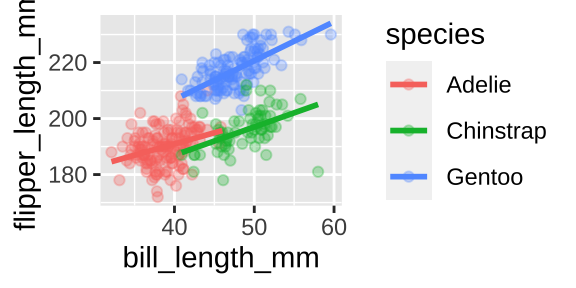We could even show these by island

ggplot(penguins, aes(x = bill_length_mm, y = flipper_length_mm, color = species)) +
geom_point(alpha = .3)+
geom_smooth(method = "lm", se = FALSE)+
facet_wrap(~island)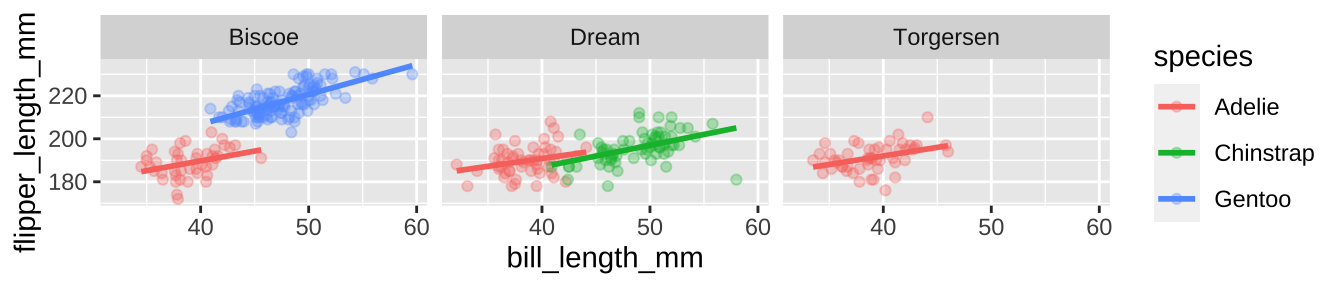## 10.4 R again Quiz

### References

Grolemund, Garrett, and Hadley Wickham. 2018. “R for Data Science.” https://r4ds.had.co.nz/.
Xie, Yihui, Joseph J Allaire, and Garrett Grolemund. 2018. R Markdown: The Definitive Guide. CRC Press.